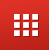Fin Shorts| February 24, 2023

# How Personal Loan Interest Rate Calculated?

Below are the factors which contribute to the calculation of Interest Rate for Personal Loan

• Interest rate: The interest rate is the main factor that determines the total interest on a personal loan. Higher the interest rate, higher the interest on the loan.
• Loan amount: The amount borrowed also impacts the interest on a personal loan. The more you borrow, the higher the interest amount will be.
• Loan term: The loan term, or the number of months over which the loan is repaid, also affects the interest. Longer loan terms generally result in higher total interest.
• Compound interest: Some personal loans may have compound interest, where interest is calculated not only on the principal but also on the accumulated interest. This can result in higher overall interest.
• Repayment frequency: The frequency of repayments also affects the total interest, with more frequent repayments typically reducing the total interest paid.
• Early repayment: If you choose to repay the loan early, it could potentially save you money on interest.

Note: It is important to understand the loan agreement and the terms and conditions for calculating interest to fully understand the total interest on a personal loan.

78
More